A more general model of cooperation based on reinforcement

learning: Alignment and Integration of the Bush-Mosteller and the

Roth-Erev model*

Paper to be presented at the Model To Model (M2M) Workshop, March 31st -  April 1st, Marseille, France.

Andreas Flache

Department of Sociology, University of Groningen

Grote Rozenstraat 31. 9712 TG  Groningen, The Netherlands

a.flache@ppsw.rug.nl

Michael W. Macy

Department of Sociology, Cornell University

Ithaca N.Y. 14853

mwm14@cornell.edu

Stochastic Collusion and the Power Law of Learning:

Aligning and Integrating the Bush-Mosteller and the Roth-Erev Reinforcement Learning Models of Cooperation

## Abstract

Analytical game theory has developed the Nash equilibrium as theoretical tool for the analysis of cooperation and conflicts in interdependent decision making. Indeterminacy and demanding rationality assumptions of the Nash equilibrium have led cognitive game theorists to explore learning-theoretic models of behavior. Two prominent examples are the Bush-Mosteller stochastic learning model and the Roth-Erev payoff-matching model. We align and integrate the two models as special cases of a General Reinforcement Learning Model. Both models predict stochastic collusion as a backward-looking solution to the problem of cooperation in social dilemmas, based on a random walk into a self-reinforcing cooperative equilibrium. The integration also uncovers hidden assumptions that constrain the generality of the theoretical derivations. Specifically, Roth and Erev assume a “Power Law of Learning” - the curious but plausible tendency for learning to diminish with success and intensify with failure, which we call “fixation.” We use computer simulation to explore the effects of fixation on stochastic collusion in three social dilemma games. The analysis shows how the integration of alternative models can uncover underlying principles and lead to a more general theory.

## 1. Introduction: Towards A Cognitive Game Theory

Conflicts as diverse as those between neighbors, friends, business associates, ethnic groups, or states, often ensue from the same fundamental logic. On the one hand the parties involved have common interests, such as avoidance of an all out nuclear war, on the other hand they may fall prey to the temptation to pursue individual agendas at the expense of the commons. To explore how and under what conditions such relationships may proceed cooperatively rather than conflictuous, game theory formalizes the problem of cooperation. At the most elementary level, the theory represents relationships as a mixed-motive two-person game with two choices, cooperate and defect. These choices intersect at four possible outcomes, abbreviated as CC, CD, DD, and DC. Each outcome has an associated payoff: R (reward), S (sucker), P (punishment) and T (temptation), respectively. Using these payoffs, we define a two-person social dilemma as any ordering of these payoffs such that mutual cooperation is Pareto optimal yet may be undermined by the temptation to cheat (if T>R) or by the fear of being cheated (if P>S) or by both. In the game of “Stag Hunt” the problem is “fear” but not “greed” (R>T>P>S), and in the game of “Chicken” the problem is “greed” but not “fear” (T>R>S>P). The problem is most challenging when both fear and greed are present, that is, when T>R and P>S. Given the assumption that R>P, there is only one way this can happen, if T>R>P>S, the celebrated game of “Prisoner’s Dilemma” (PD).

The Nash equilibrium - the main solution concept in analytical game theory - predicts mutual defection in PD, unilateral defection in Chicken, and either mutual cooperation or mutual defection in Stag Hunt. However, Nash cannot make precise predictions about the selection of supergame equilibria, that is, about the outcome of on-going mixed-motive games. Nor can it tell us much about the dynamics by which a population of players can move from one equilibrium to another. These limitations, along with concerns about the cognitive demands of forward-looking rationality (Dawes and Thaler 1988; Weibull 1998; Fudenberg and Levine 1998), have led game theorists to explore models of cognition that explicitly describe the dynamics of stepwise decision making.  This is reflected in a growing number of formal learning-theoretic models of cooperative behavior (Macy 1991; Roth and Erev 1995; Fudenberg and Levine 1998; Peyton-Young 1998; Cohen et. al. 2001). In learning, positive outcomes increase the probability that the associated behavior will be repeated, while negative outcomes reduce it.

In general form, these simple game-theoretic learning models consist of a probabilistic decision rule and a learning algorithm in which game payoffs are evaluated relative to an aspiration level, and the corresponding choice propensities are updated accordingly. The schematic model is diagrammed in Figure 1.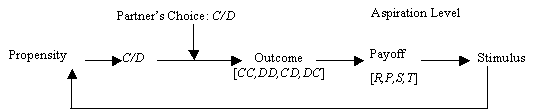Figure 1. Schematic model of reinforcement learning.

The first step in Figure 1 is the decision by each player whether to cooperate or defect. This decision is probabilistic, based on the player’s current propensity to cooperate. The resulting outcome then generates payoffs (R, S, P, or T) that the players evaluate as satisfactory or unsatisfactory relative to their aspiration level. Satisfactory payoffs present a positive stimulus (or reward) and unsatisfactory payoffs present a negative stimulus (or punishment). These rewards and punishments then modify the probability of repeating the associated choice, such that satisfactory choices become more likely to be repeated, while unsatisfactory choices become less likely.

Erev, Roth and others (Roth and Erev 1995; Erev and Roth 1998; Erev et al. 1999) used a learning model to estimate globally applicable parameters from data collected across a variety of human subject experiments. They concluded that “low rationality” models of reinforcement learning may often provide a more accurate prediction than orthodox game theoretical analysis. Macy (1995) and Flache (1996) have also tested a simple reinforcement learning algorithm in controlled social dilemma experiments and found supporting evidence.

Learning models have also been applied outside the laboratory to mixed-motive games played in everyday life, in which cooperation is largely unthinking and automatic, based on heuristics, habits, routines, or norms, such as the propensity to loan a tool to a neighbor, tell the truth, or trouble oneself to vote. For example, Kanazawa (2000) used voting data to show how a simple learning model suggested by Macy (1991) solves the “voter paradox” in public choice theory.

While interest in cognitive game theory is clearly growing, recent studies have been divided by disciplinary boundaries between sociology, psychology, and economics that have obstructed the theoretical integration needed to isolate and identify the learning principles that underlie new solution concepts. Our paper aims to move towards such an integration. We align two prominent models in cognitive game theory and integrate them into a general model of adaptive behavior in two-person mixed-motive games (or social dilemmas). In doing so, we will identify a set of model-independent learning principles that are necessary and sufficient to generate cooperative solutions. We begin by briefly summarizing the basic principles of reinforcement learning and then introduce two formal specifications of these principles, the Bush-Mosteller stochastic learning model and the Roth-Erev payoff matching model.

1.1 Learning Theory and the Law of Effect

In reinforcement learning theory, the search for solutions is “backward-looking” (Macy 1990) in that it is driven by experience rather than the forward-looking calculation assumed in analytical game theory (Fudenberg and Levine 1998; Weibull 1998). Thorndike (1898) formulated this as the “Law of Effect,” based on the cognitive psychology of William James. If a behavioral response has a favorable outcome, the neural pathways that triggered the behavior are strengthened, which “loads the dice in favor of those of its performances which make for the most permanent interests of the brain’s owner” (James 1981, p. 143). This connectionist theory anticipates the error back-propagation used in contemporary neural networks (Rummelhart and McLelland 1988). These models show how highly complex behavioral responses can be acquired through repeated exposure to a problem. For example, with a little genetic predisposition and a lot of practice, we can learn to catch a ball while running at full speed, without having to stop and calculate the trajectory (as the ball passes overhead).

More precisely, learning theory relaxes three key behavioral assumptions in analytical game-theoretic models of decision:

·         Propinquity replaces causality as the link between choices and payoffs. Learning theory assumes experiential induction rather than logical deduction. Players explore the likely consequences of alternative choices and develop preferences for those associated with better outcomes, even though the association may be coincident, “superstitious,” or causally spurious.

·         Reward and punishment replace utility as the motivation for choice. Learning theory differs from game-theoretic utility theory in positing two distinct cognitive mechanisms that guide decisions toward better outcomes, approach (driven by reward) and avoidance (driven by punishment). The distinction means that aspiration levels are very important for learning theory. The effect of an outcome depends decisively on whether it is coded as gain or loss, satisfactory or unsatisfactory, pleasant or aversive.

·         Melioration replaces optimization as the basis for the distribution of choices over time. Melioration refers to suboptimal gradient climbing when confronted with “distributed choice” (Herrnstein and Drazin 1991) across recurrent decisions. Melioration implies a tendency to repeat choices with satisfactory outcomes even if other choices have higher utility, a behavioral tendency March and Simon (1958) call “satisficing.” In contrast, unsatisfactory outcomes induce search for alternative outcomes, including a tendency to revisit alternative choices whose outcomes are even worse, a pattern we call “dissatisficing.”

The Law of Effect does not solve the social dilemma, it merely reframes it: Where the penalty for cooperation is larger than the reward, and the reward for aggressive behavior is larger than the penalty, how can penalty-aversive, reward-seeking actors elude the trap of mutual punishment?

1.2 The Bush-Mosteller Stochastic Learning Model

The earliest answer was given by Rapoport and Chammah (1965), who used learning theory to propose a Markov model of Prisoner’s Dilemma with state transition probabilities given by the payoffs for each state, based on the assumption that each player is satisfied only when the partner cooperates. With choice probabilities updated after each move based on the Law of Effect, mutual cooperation is an absorbing state in the Markov chain.

Macy (1990, 1991) elaborated Rapoport and Chammah’s analysis using computer simulations of their Bush-Mosteller stochastic learning model. Macy identified two learning-theoretic equilibria in the PD game, corresponding to each of the two learning mechanisms, approach and avoidance. Approach implies a self-reinforcing equilibrium (SRE) characterized by satisficing behavior. The SRE obtains when a strategy pair yields payoffs that are mutually rewarding. The SRE can obtain even when both players receive less than their optimal payoff (such as the R payoff for mutual cooperation in the PD game), so long as this payoff exceeds aspirations. Avoidance implies an aversive self-correcting equilibrium (SCE) characterized by dissatisficing behavior. Dissatisficing means that both players will try to avoid an outcome that is better than their worst possible payoff (such as P in the PD game), so long as this payoff is below aspirations. The SCE obtains when the expected change of probabilities is zero and there is a positive probability of punishment as well as reward. This happens when outcomes that reward cooperation or punish defection (causing the probability of cooperation to increase) balance outcomes that punish cooperation or reward defection (causing the probability to decline). At equilibrium, the dynamics pushing the probability higher are balanced by the dynamics pushing in the other direction, like a tug-of-war between two equally strong teams.

These learning theoretic equilibria differ fundamentally from the Nash predictions in that agents have an incentive to unilaterally change strategy. In the SRE, both players have an incentive to deviate from unconditional mutual cooperation, but they stay the course so long as the R payoff exceeds their aspirations. Unlike the Nash equilibrium, the SCE has players constantly changing course, but their efforts are self-defeating. It is not that everyone decides they are doing the best they can, it is that their efforts to do better set into motion a dynamic that pulls the rug out from under everyone.

Suppose two players in PD are each satisfied only when the partner cooperates, and each starts out with zero probability of cooperation. They are both certain to defect, which then causes both probabilities to increase (as an avoidance response). Paradoxically, an increased probability of cooperation now makes a unilateral outcome (CD or DC) more likely than before, and these outcomes punish the cooperator and reward the defector, causing both probabilities to drop. Nevertheless, there is always the chance that both players will defect anyway, causing probabilities to rise further still. Once both probabilities exceed 0.5, further increases become self-reinforcing, by increasing the chances for another bilateral move, and this move is now more likely to be mutual cooperation instead of mutual defection. In short, the players can escape the social trap through stochastic collusion, characterized by a chance sequence of bilateral moves in a “drunkard’s walk.” A fortuitous string of bilateral outcomes can increase cooperative probabilities to the point that cooperation becomes self-sustaining - the drunkard wanders out of the gully.

1.3 The Roth-Erev Payoff-Matching Model

More recently, Roth and Erev (Roth and Erev 1995; Erev and Roth 1998; Erev et al. 1999) have proposed a learning-theoretic alternative to the earlier Bush-Mosteller formulation. Their model draws on the “matching law” which holds that adaptive actors will choose between alternatives in a ratio that matches the ratio of reward. Applied to social dilemmas, the matching law predicts that players will learn to cooperate to the extent that the payoff for cooperation exceeds that for defection, which is possible only if both players happen to cooperate and defect at the same time (given R>P). As with Bush-Mosteller, the path to cooperation is a sequence of bilateral moves.

Like the Bush-Mosteller stochastic learning model, the Roth-Erev payoff matching model implements the three basic principles that distinguish learning from utility theory – experiential induction (vs. logical deduction), reward and punishment (vs. utility), and melioration (vs. optimization). The similarity in substantive assumptions makes it tempting to assume that the two models are mathematically equivalent, or if not, that they nevertheless give equivalent solutions.

On closer inspection, however, we find important differences. Each specification implements reinforcement learning in different ways, and with different results. Without a systematic theoretical alignment and integration of the two algorithms, it is not clear whether and under what conditions the backward-looking solution for social dilemmas identified with the Bush-Mosteller (BM) specification generalizes to the Roth-Erev (RE) model.

Those assumptions can be brought to the surface by close comparison of competing models and by integrating alternative specifications as special cases of a more general model. This is especially important for models that must rely on computational rather than mathematical methods. “Without such a process of close comparison, computational modeling will never provide the clear sense of ‘domain of validity’ that typically can be obtained for mathematized theories” (Axtell et al. 1996, p. 123).

Unfortunately, learning models have been divided by disciplinary boundaries between sociology, psychology, and economics that have obstructed the theoretical integration needed to isolate and identify the learning principles that underlie new solution concepts. Accordingly, this paper aims to align two prominent models in cognitive game theory and to integrate them into a general model of adaptive behavior in two-person mixed-motive games. By “docking” (Axtell et al. 1996) the BM stochastic learning model with the RE payoff-matching model, we can identify learning principles that generalize beyond particular specifications, and at the same time, uncover hidden assumptions that explain differences in the outcomes and constrain the generality of the theoretical derivations.

In section 2 that follows, we formally align the two learning models and integrate them into a General Reinforcement Learning (GRL) model, for which the Bush-Mosteller and Roth-Erev models are special cases. This process brings to the surface a key hidden assumption with important implications for the generality of learning-theoretic solution concepts. Section 3 then uses computer simulations of the integrated model to explore the effects of model differences across a range of two-person social dilemma games. The analyses confirm the generality of stochastic collusion but also show that its determinants depend decisively on whether BM or RE assumptions about learning dynamics are employed.

## 2. The Bush-Mosteller and Roth-Erev Specifications

In general form, both the BM and RE models implement the stochastic decision process diagrammed in Figure 1, in which choice propensities are updated by the associated outcomes. Thus, both models imply the existence of some aspiration level relative to which cardinal payoffs can be positively or negatively evaluated. Formally, the stimulus s associated with action a is calculated as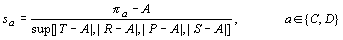where pa is the payoff associated with action a (R or S if = C, and T or P if = D) and sa is a positive or negative stimulus derived from pa. The denominator in  represents the upper value of the set of possible differences between payoff and aspiration. With this scaling factor, stimulus s is always equal to or less than unity in absolute value, regardless of the magnitude of the corresponding payoff.

Neither model imposes constraints on the determinants of aspirations. Whether aspirations are high or low or habituate with experience depends on assumptions that are exogenous to both models.

2.1 Aligning the two models

The two models diverge at the point that these evaluations are used to update choice probabilities. The Bush-Mosteller stochastic learning algorithm updates probabilities following an action a (cooperation or defection) as follows: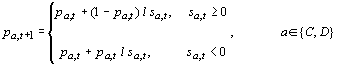In equation , pa,t is the probability of action a at time t and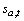is the positive or negative stimulus given by . The change in the probability for the action not taken, b, obtains from the constraint that probabilities always sum to one, i.e.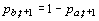. The parameter l is a constant (0 < l < 1) that scales the learning rate. With l » 0, learning is very slow, and with » 1, the model approximates a “win-stay, lose-shift” strategy (Catania 1992).

For any value of l, Equation 2 implies a decreasing effect of reward as the associated propensity approaches unity, but an increasing effect of punishment. Similarly, as the propensity approaches zero, there is a decreasing effect of punishment and a growing effect of reward. This constrains probabilities to approach asymptotically their natural limits.

Like the Bush-Mosteller, the Roth-Erev model is stochastic, but the probabilities are not equivalent to propensities. Propensities are a function of cumulative satisfication and dissatisfaction with the associated choices, and probabilities are a function of the ratio of propensities. More precisely, the propensity q for action a at time T is the sum of all stimuli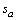a player has ever received when playing a: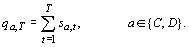Roth and Erev then use a “probabilistic choice rule” to translate propensities into behavior. The probability pa of action a at time t+1 is the propensity for a divided by the sum of the propensities at time t: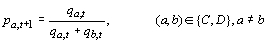where a and b represent the binary choices to cooperate or defect. Following action a, the associated propensity qa increases if the payoff is positive relative to aspirations (by increasing the numerator in ) and decreases if negative. The propensity for b remains constant, but the probability of b declines (by increasing the denominator in the equivalent expression for pb,t+1).

An obvious problem with the specification of equations  and  (but not ) is the possibility of negative probabilities if punishment dominates reinforcement. In their original model, Erev, Roth and Bereby-Meyer (1999) circumvent this problem with the ad hoc addition of a “clipping” rule. More precisely, their implementation assumes that the new propensity qa,t+1 = qa,t + sa,t  except for the case where this sum drops below a very small positive constant n. In that case, the new propensity is reset to n. Equations  and  represent the RE clipping rule by rewriting  as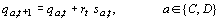where a is the action taken in round t (either cooperation or defection) and r is a response function of the form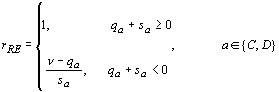where all terms except n are indexed on t. This solution causes the response to reinforcement to become discontinuous as propensities approach the lower bound n. In order to align the model with Bush-Mosteller, we replaced the discontinuous function in  with a more elegant solution. Equation  gives asymptotic lower as well as upper limits to the probabilites: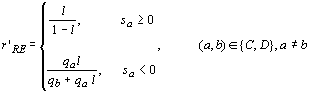where all terms except l (the constant learning rate) are indexed on t. The parameter l sets the baseline learning rate between zero and one (as in ), rather than leaving l implied by the relative magnitude of the payoffs (as in ). More importantly,  aligns Roth-Erev with Bush-Mosteller by eliminating the need for a theoretically arbitrary clipping rule. For sa ³ 0,  is equivalent to  except that rewards are multiplied by the constant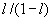that increases exponentially with the learning rate l. The important change is for the case where sa < 0. Now r is decreasing in qa (with a limit at zero). Hence, the marginal effect of additional punishment for action a approaches zero as the corresponding propensity approaches zero.

Equation  aligns Roth-Erev with Bush-Mosteller, allowing easy identification of the essential difference, which is inscribed in equation . Rewards increase the denominator in , depressesing the effect on probabilities of unit changes in the numerator. Punishments have the opposite effect, allowing faster changes in probabilities.

To see the significance of this difference, consider first the special case where learning is based on rewards of different magnitude and no stimuli are aversive. Here the RE model corresponds to Blackburn’s (1936) "Power Law of Practice.” Erev and Roth (1998) cite Blackburn to support the version of their model that precludes punishment, such that “learning curves tend to be steep initially and then flatten" (1998:859). In the version with both reward and punishment, their model implies what might be termed a “Power Law of Learning,” in which responsiveness to stimuli declines exponentially with reward and increases with punishment. We have no knowledge of a Power Law of Learning or of any corresponding learning-theoretic concept. For convenience, we have borrowed “fixation” from Freudian psychology.

Fixation is the principal difference between the BM and RE models. Unlike the BM model, which precludes fixation, the RE model builds this in as a necessary implication of the learning algorithm in . The two models make convergent predictions about:

• the declining marginal impact of repeated reinforcement on the response to yet another reward.
• the increasing marginal impact of repeated punishment on the response to an occasional reward.

However, the two models make competing predictions about the effects of repeated punishment on the response to additional punishment, and on the effects of repeated reward on the response to an occasional punishment, as summarized in Table 1. Bush-Mosteller assumes that the marginal impact of punishment decreases with repetition, while punishment has its maximum effect following repeated reward for a given action. The Power Law of Learning implies quite the opposite. Repeated failure arouses attention and restores an interest in learning. Hence, the marginal impact of punishment increases with punishment. Conversely, repeated success leads to complacency and inattention to the consequences of behavior, be it reward or punishment.

 Following Repeated Reinforcement of C: Following Repeated Punishment of C: Response to Reward Response to Punishment Response to Reward Response to Punishment of C of D of C of D of C of D of C of D BM Decreases Increases Increases Decreases Increases Decreases Decreases Increases RE Decreases Decreases Decreases Decreases Increases Increases Increases Increases

Table 1. Change in response to reward and punishment following a recurrent stimulus.

Note that fixation as implemented in the RE learning model should not be confused with either satisficing or habituation, two well-known behavioral mechanisms that can also promote the routinization of behavior. Fixation differs from satisficing in both the causes and effects. Satisficing is caused by a sequence of reinforcements that are associated with a given behavior, which causes the probability of choosing that behavior to approach unity, thereby precluding the chance to find a better alternative. Fixation is caused by a sequence of reinforcements for any behavior. Thus, fixation “fixes” any probability distribution over a set of behaviors, including indifference, so long as all behaviors are rewarded. Satisficing and fixation also differ in their effects. Satisficing inhibits search but has no effect on responsiveness to reinforcement. Fixation does not preclude search but instead inhibits responses to the outcomes of all behaviors that may be explored.

Fixation also differs from habituation, in both the causes and effects. Habituation is caused by repeated presentation of a stimulus, be it a reward or a punishment. Fixation is caused only by repeated reinforcement; repeated punishment disrupts fixation and restores learning. The effects also differ. Habituation to reward increases sensitivity to punishment, while fixation inhibits responsiveness to both reward and punishment.

2.2 Integrating the two models

To suppress fixation, Roth and Erev (1995; see also Erev and Roth 1998) added discontinuous functions for “forgetting,” which suddenly resets the propensity to some lower value. Like the “clipping” rule, the “forgetting” rule is theoretically ad hoc and mathematically inelegant. Equation  offers a more elegant solution that permits continuous variation in the level of fixation, parameterized as f: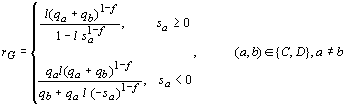As in , all parameters except f and l are time indexed. The parameter f represents the level of fixation, 0 £ f £ 1. Equation  is identical to  in the limiting case where f = 1, corresponding to the RE model which fixation hardwired.

But notice what happens when f = 0. Equation  now reduces to the BM stochastic learning algorithm! (The proof is elaborated in the Appendix.). By replacing the discontinuous “clipping” and “forgetting” functions used by Roth-Erev with continuous functions, we arrive at a General Reinforcement Learning (GRL) model, with a smoothed version of Roth-Erev and the original Bush-Mosteller as special cases. Hence, the response function in  is expressed as rG, denoting the generality of the model. Bush-Mosteller and Roth-Erev are now fully aligned and integrated.

## 3. A General Theory of Cooperation in Social Dilemmas

The GRL model in equations , , , and  includes three parameters - aspiration level (A), learning rate (l), and fixation (f) - that can be manipulated to study each of the four mechanisms that we have identified as elements of a learning-theoretic solution concept for social dilemmas: satisficing, dissatisficing, fixation, and random walk. The model allows us to independently manipulate satisficing (precluded by high aspirations), dissatisficing (precluded by low aspirations), fixation (precluded by a fixed learning rate), and random walk (precluded by a low learning rate). We can then systematically explore the solutions that emerge with different parameter combinations over each of the three classic types of social dilemma.

3.1 The Baseline Model: Stochastic Collusion in Social Dilemmas

We begin by using the GRL model to replicate findings in previous research with the BM specification. This provides a baseline for comparing the learning dynamics in the RE model, specifically, the effects of fixation in interaction with aspiration levels and learning rates.

Macy and Flache (2002) used the BM model to study learning dynamics in three characteristic types of two-person social dilemma games, PD, Chicken, and Stag Hunt. They idenfied a socially deficient SCE in all three social dilemmas. We can compute SCE analytically by finding the level of cooperation at which the expected change in the probability of cooperation is zero. The expected change is zero when for both players the probability of outcomes that reward cooperation or punish defection, weighted by the absolute value of the associated stimuli, equals the probability of outcomes that punish cooperation or reward defection, weighted likewise. With A = 2 and the payoff vector [4,3,1,0], the SCE occurs at pc = 0.37 in PD and at pc = 0.5 in Chicken and Stag Hunt, respectively. Across all possible payoffs, the equilibrium cooperation rate in PD is always below pc = 0.5, which is the asymptotic upper bound that the solution approaches as R approaches T and P approaches S simultaneously. The lower bound is pc = 0 as P approaches A. In Chicken, the corresponding upper bound is pc = 1 as R approaches T and S approaches A. The lower bound is pc = 0 as R, S, and P all converge on A (retaining R>A>S>P). Only in Stag Hunt is it possible that there is no SCE, if R-T > A-S. The lower bound for Stag Hunt is pc = 0 as T approaches R and P approaches A.

Using computer simulation, Macy and Flache (2002) show that it is possible to escape this equilibrium through random walk not only in Prisoner’s Dilemma but in all dyadic social dilemma games. However, the viability of the solution critically depends on actors’ aspiration levels, i.e. the benchmark that distinguishes between satisfactory and unsatisfactory outcomes. A self-reinforcing cooperative equilibrium is possible if and only if both players’ aspiration levels are lower than the payoff for mutual cooperation (R). Aspirations above this point necessarily preclude a learning theoretic solution to social dilemmas.

Very low aspirations do not preclude mutual cooperation as an equilibrium but may prevent adaptive actors from finding it. If aspiration levels are below maximin, then mutual or unilateral defection may also be a self-reinforcing equilibrium, even though these outcomes are socially deficient. Once the two players stumble into one of these outcomes, there is no escape, so long as the outcome is mutually reinforcing. If the aspiration level exceeds maximin and falls below R, there is a unique SRE in which both players receive a reward, namely, mutual cooperation.

If there is at least one SRE in the game matrix, then escape from the SCE is inevitable. It is only a matter of time until a chance sequence of moves causes players to lock-in a mutually reinforcing combination of strategy choices. However, the wait may not be practical in the intermediate term if the learning rate is very low. The lower the learning rate, the larger the number of steps that must be fortuitously coordinated to escape the “pull” of the SCE. Thus, the odds of attaining lock-in increase with the step-size in a random walk (see also Macy 1989, 1991).

To summarize, the BM stochastic learning model identifies a social trap - a socially deficient SCE - that lurks inside every PD and Chicken game and most games of Stag Hunt. The model also identifies a backward-looking solution, mutually reinforcing cooperation. However, this solution obtains only when both players have aspiration levels below R, such that each is satisfied when the partner cooperates. Stochastic collusion via random walk is possible only if aspirations are also above maximin and is viable for the intermediate term only if the learning rate is sufficiently high.

We can test whether these Bush-Mosteller results can be replicated with the GRL model by setting parameters to preclude fixation and to allow random walk, satisficing, and dissatisficing. More precisely:

·         Fixation is precluded by setting f = 0.

·         Aspirations are fixed midway between maximin and minimax. With the payoffs ordered from the set [4,3,1,0] for each of the three social dilemma payoff inequalities, minimax is always 3, maximin is always 1, and A = 2. This aspiration level can be interpreted as the expected payoff when behavioral propensities are uninformed by prior experience (pa = 0.5) such that all four payoffs are equiprobable.This means that mutual cooperation is a unique SRE in each of the three social dilemma games.

·         We set the baseline learning rate l high enough to facilitate random walk into the SRE at CC (l = 0.5).

Figure 2 confirms the possibility of stochastic collusion in all three social dilemma games, assuming moderate learning rates, moderate aspirations, and no fixation. The figure charts the change in the probability of cooperation, pc for one of two players with statistically identical probabilities.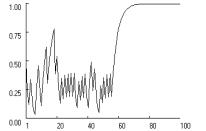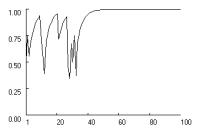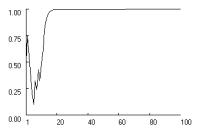Prisoner’s Dilemma (T>R>A>P>S) Chicken (T>R>A>S>P) Stag Hunt (R>T>A>P>S) Figure 2. Stochastic collusion in three social dilemma games. (p = [4,3,1,0], A = 2, l = 0.5, f = 0, qc,1 = qd,1 = 1.

Figure 2 shows how dissatisficing players wander about in a SCE with a positive probability of cooperation that eventually allows them to escape the social trap by random walk. With = 0, the general model reproduces the characteristic Bush-Mosteller pattern of stochastic collusion in all three games, but not with equal probability. Mutual cooperation locks in most readily in Stag Hunt and least readily in Prisoner’s Dilemma. To test the robustness of this difference, we simulated 1000 replications of this experiment and measured the proportion of runs that locked into mutual cooperation within 250 iterations. The results confirm the differences between the games. In Prisoner’s Dilemma, the lock-in rate for mutual cooperation was only 0.66, while it was 0.96 in Chicken and 1.0 in Stag Hunt.

These differences reflect subtle but important interactions between aspiration levels and the type of social dilemma - the relative importance of fear (the problem in Stag Hunt) and greed (the problem in Chicken). The simulations also show that satisficing is equally important, at least in the Prisoner’s Dilemma and in the Chicken Game. In these games, appreciation that the payoff for mutual cooperation is “good enough” motivates players to stay the course despite the temptation to cheat (given T>R). Otherwise, mutual cooperation will not be self-reinforcing. In Stag Hunt, satisficing is less needed in the long run, because there is no temptation to cheat (R>T). However, despite the absence of greed, the simulations reveal that, even in Stag Hunt, fear may inhibit stochastic collusion if high aspirations limit satisficing. Simulations confirmed that, in the absence of fixation, there is an optimal balance point for the aspiration level between satisficing and dissatisficing and between maximin and R.

3.2 Effects of fixation on stochastic collusion

With the Bush-Mosteller dynamics as a baseline, we now systematically explore the effects of fixation in interaction with the learning rate and aspirations. Knowledge of these effects will yield a general theory of the governing dynamics for reinforcement learning in social dilemmas, of which BM and RE are special cases.

Fixation has important implications for the effective learning rate, which in turn influences the coordination complexity of stochastic collusion. In both the BM and revised RE models, the effective learning rate depends on the baseline rate (l) and the magnitude of p. Even if the baseline learning rate is high, the effective rate approaches zero as p asymptotically approaches the natural limits of probability. The RE model adds fixation as an additional determinant of effective learning rates. Fixation implies a tendency for learning to slow down with success, and this can be expected to stabilize the SRE. Even if one player should occasionally cheat, fixation prevents either side from paying much attention. Thus, cooperative propensities remain high, and the rewards that induce fixation are quickly restored.

Fixation also implies that learning speeds up with failure. This should destabilize the SCE, leading to a higher probability of random walk into stochastic collusion, all else being equal. This effect of fixation should be even stronger when aspirations are high. The higher actors’ aspirations, the smaller are the rewards and the larger the punishments that players’ can experience. As aspirations approach the R payoff (from below), punishments may become so strong that “de-fixation” propels actors into stochastic collusion following a single incidence of mutual defection.

The lower actors’ aspirations, the larger are the rewards and the smaller the punishments that players’ can experience. Fixation on reward then reduces the effective learning rate, thereby increasing the coordination complexity of random walk into the SRE. The smaller the step size, the longer it takes for random walk out of the SCE.

Simply put, when aspirations are above maximin, the Power Law of Learning implies a higher likelihood of obtaining a cooperative equilibrium in social dilemmas, compared to what would be expected in the absence of a tendency toward fixation, all else being equal. However, the opposite is the case when aspirations are low.

We used computational experiments to test these intuitions about how fixation interacts with learning rates and aspirations to alter the governing dynamics in each of three types of social dilemma, using a two (learning rates: high/low) by two (fixation: high/low) by two (aspirations: high/low) factorial design, with fixation and aspiration levels nested within learning rates. Based on the results for the BM model (with f = 0), we set the learning rate l at a level high enough to facilitate stochastic collusion (0.5) and low enough to preclude stochastic collusion (0.05). We begin with a high learning rate in order to study the effects of fixation on the attraction and stability of the SRE. We then use a low learning rate to look at the effects on the stability of socially deficient SCE.

Within each learning rate, we manipulated fixation using two levels, = 0, corresponding to the BM assumption and f = 0.5 as an approximation of the RE model with a moderate level of fixation. (For robustness, we also tested f = 1 but found no qualitative difference with moderate fixation.) Within each level of fixation, we manipulated the aspiration level below and above A = 2.0 to see how fixation affects the optimal aspiration level for random walk into mutual cooperation.

We begin by testing the interaction between fixation and satisficing by setting aspirations close to the lower limit at maximin. With A = 1.05 and l = 0.5, mutual cooperation is the unique SRE in all three games, and stochastic collusion is possible even without fixation. Low aspirations increase the tendency to satisfice, making it more difficult to escape a socially deficient SCE. With low aspirations, the predominance of reward over punishment means that fixation can be expected to increase the difficulty by further stabilizing the SCE.

This intuition is confirmed in Figure 3 across all three games. The dotted lines show the cooperation rate with fixation (f = 0.5) and the solid line shows the rate without fixation. Without fixation, low aspirations delay lock-in on mutual cooperation relative to the moderate aspirations in the baseline condition (see Figure 2), but lock-in is still possible within the first 100 iterations. For reliability, we measured the proportion of runs that locked in mutual cooperation within 250 iterations, based on 1000 replications with A = 1.05. We found that in the Prisoner’s Dilemma and in the Chicken Game, the lower aspiration level caused a significant decline in the rate of mutual cooperation relative to the baseline condition, but it did not suppress cooperation entirely. In the Prisoner’s Dilemma, the cooperation rate declined from 0.66 in the baseline condition to about 0.14, and for the Chicken Game the corresponding reduction was from 0.96 to 0.83. Only in Stag Hunt did lower aspirations not affect the rate of mutual cooperation.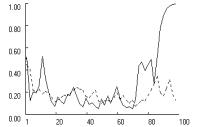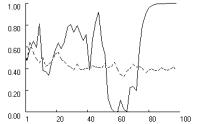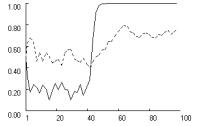Prisoner’s Dilemma (T>R>A>P>S) Chicken (T>R>A>S>P) Stag Hunt (R>T>A>P>S) Figure 3. Effect of low aspirations on cooperation rates, with and without fixation (dotted  and solid) in three social dilemmas (p = [4,3,1,0], A = 1.05, l = 0.5, f = [0, 0.5], qc,1 = qd,1 = 1).

As expected, fixation considerably exacerbates the problem caused by low aspirations. Figure 3 shows how with = 0.5, lock-in fails to obtain entirely in the Prisoner’s Dilemma and Chicken, and is significantly delayed in Stag Hunt. Reliability tests reveal a highly significant effect of fixation. Based on 1000 replications with = 0.5, the rate of lock-in within 250 iterations dropped to zero in all three games.

With high aspirations, fixation should have the opposite effect. Without fixation, high aspirations increase the tendency to dissatisfice (over-explore), which should destabilize the SRE. However, the predominance of punishment over reward means that “de-fixation” can be expected to promote cooperation by negating the destabilizing effects of dissatisficing. This intuition is confirmed in Figure 4. For symmetry with Figure 3, we assumed an aspiration level of = 2.95, just below the minimax payoff. Without fixation, stochastic collusion remains possible but becomes much more difficult to attain, due to the absence of satisficing.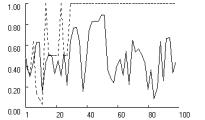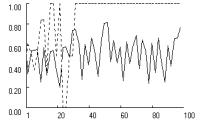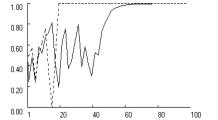Prisoner’s Dilemma (T>R>A>P>S) Chicken (T>R>A>S>P) Stag Hunt (R>T>A>P>S) Figure 4. Effect of high aspirations on cooperation rates, with and without fixation (dotted and solid) in three social dilemmas (p = [4,3,1,0], A = 2.95, l = 0.5, f = [0, 0.5], qc,1 = qd,1 = 1).

Figure 4 reveals an effect of fixation that is similar across the three payoff structures. Without fixation, high aspirations preclude stochastic collusion in Prisoner’s Dilemma and Chicken. In Stag Hunt, mutual cooperation remains possible but is delayed relative to the baseline condition in Figure 2. With high aspirations, fixation causes the effective learning rate to increase within the first 20 or so iterations, up to a level that is sufficient to quickly obtain lock-in on mutual cooperation. Reliability tests show that the pattern is highly robust. Based on 1000 replications without fixation, the rate of stochastic collusion within 250 iterations was zero in Prisoner’s Dilemma and Chicken, and 0.64 in Stag Hunt. With fixation (f = 0.5), this rate increased to nearly one in all three games (0.997, 0.997 and 0.932 in PD, Chicken and Stag Hunt, respectively).

To further test the interaction between aspirations and fixation, we varied the aspiration level A across the entire range of payoffs (from 0 to 4) in steps of 0.1. Figure 5 reports the effect of aspirations on the rate of stochastic collusion within 250 iterations, based on 1000 replications with (dotted line) and without (solid line) fixation.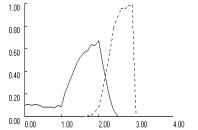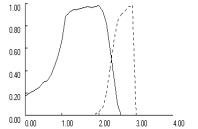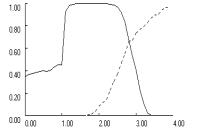Prisoner’s Dilemma (T>R>P>S) Chicken (T>R>S>P) Stag Hunt (R>T>P>S) Figure 5. Effects of aspiration level on stochastic collusion within 250 iterations, with and without fixation (dotted and solid) in three social dilemmas (p = [4,3,1,0], l = 0.5, f = [0, 0.5], qc,1 = qd,1 = 1, N = 1000).

Figure 5 clearly demonstrates the interaction effects. In all three games, fixation reduces cooperation at low aspiration levels and increases cooperation at high aspiration levels. Fixation also shifts the optimal balance point for aspirations to a level close to the R payoff of the game, or A = 3 in PD and Chicken and A = 4 in Stag Hunt. In addition, the figure reveals that the interaction between fixation and low aspirations does not depend on whether aspirations are below or above the maximin payoff. As long as aspirations levels fall below A = 2, moderate fixation (f = 0.5) suppresses random walk into mutual cooperation.

However, the mechanisms differ, depending on whether aspirations are above or below maximin. Below maximin, the predominant self-reinforcing equilibria are deficient outcomes, mutual defection in the Prisoner’s Dilemma and Stag Hunt and unilateral cooperation in Chicken. These equilibria prevail at low aspiration levels because their coordination complexity is considerably lower than that of mutual cooperation. Accordingly, fixation reduces cooperation in this region because it increases the odds that learning dynamics converge on the SRE that is easiest to coordinate, at the expense of mutual cooperation. With aspirations levels above maximin, fixation no longer induces convergence on a deficient SRE, but it inhibits convergence on the unique SRE of mutual cooperation.

To sum up, the GRL model shows that stochastic collusion is a fundamental solution concept for all social dilemmas. However, we also find that the viability of stochastic collusion depends decisively on the assumptions about fixation. With high aspirations, fixation makes stochastic collusion more likely, while with low aspirations, it makes cooperation more difficult.

3.3 Self-correcting equilibrium and fixation

We now use low learning rates to study the effect of fixation on the stability of the socially deficient SCE. The coordination complexity of random walk increases exponentially as the learning rate decreases. In our baseline condition with A = 2 and f = 0, but a low learning rate of l = 0.05, stochastic collusion is effectively precluded in all three social dilemma games, even after 1000 iterations and with moderate aspiration levels. We confirmed this by measuring mean cooperation in the 1000th iteration over 1000 replications. For all three types of social dilemma, the mean was not statistically different from the SCE derived analytically from the payoffs (p = 0.366 for PD and p = 0.5 for Chicken and Stag Hunt).

We want to know if fixation makes it easier to escape and whether this depends on the level of aspirations. To find out, we crossed fixation (f = 0 and f = 0.5) with aspirations just above maximin (= 1.05) and just below minimax (= 2.95), just as we did in the previous study of stochastic collusion with high learning rates.

When aspirations are low, the predominance of reward over punishment should cause the effective learning rate to decline as players fixate on repeated reward. With the baseline learning rate already near zero, fixation should have little effect, and this is confirmed in Figure 6, based on 1000 iterations in each of the three games. Reduction in the effective learning rate merely smooths out fluctuations in players’ probability of cooperation, pc , around an equilibrium level of about pc = 0.13 in the Prisoner’s Dilemma, and pc = 0.5 in Chicken and Stag Hunt, respectively. Reliability tests showed a slight negative effect of fixation on the equilibrium rate of cooperation. Based on 1000 replications, we found that in the PD and Chicken, the rate of stochastic collusion within 1000 iterations was zero, with or without fixation. In Stag Hunt, stochastic collusion remained possible without fixation (with a rate of 0.21), but this rate dropped to zero with f = 0.5. In short, without fixation, the low learning rate of = 0.05 allows players to escape the SCE only in Stag Hunt. Fixation suppresses even this possibility.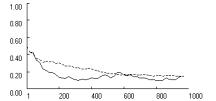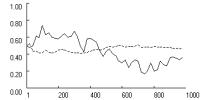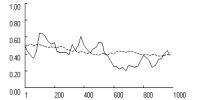Prisoner’s Dilemma (T>R>A>P>S) Chicken (T>R>A>S>P) Stag Hunt (R>T>A>P>S) Figure 6.     Effects of fixation (dotted line) on SCE with low learning rates and low aspirations in three social dilemma games (p = [4,3,1,0], l = 0.05,  A = 1.05, f = [0, 0.5], qc,1 = qd,1 = 1).

With high aspirations - and a predominance of punishment over reward - fixation should have the opposite effect, causing the effective learning rate to increase. This in turn should make stochastic collusion a more viable solution. To test this possibility, we increased the aspiration level to A = 2.95.

Figure 7 confirms the expected cooperative effect of fixation when aspirations are high. The increase in the effective learning rate helps players escape the SCE within about 250 iterations in all three games. Reliability tests show a very powerful effect of fixation. With f = 0, the rate of stochastic collusion within 1000 iterations is zero in all three games. With moderate fixation (f = 0.5), stochastic collusion within 1000 iterations becomes virtually certain in all three games.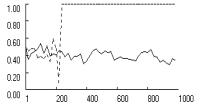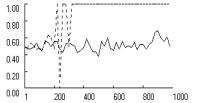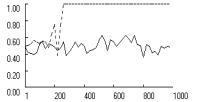Prisoner’s Dilemma (T>R>A>P>S) Chicken (T>R>A>S>P) Stag Hunt (R>T>A>P>S) Figure 7.     Effects of fixation (dotted line) on SCE with low learning rates and high aspirations, in three social dilemma games (p = [4,3,1,0], l = 0.05, A = 2.95, f = [0, 0.5], qc,1 = qd,1 = 1).

As an additional test, we varied the aspiration level A from 0 to 4 in steps of 0.1, exactly as in Figure 5, only this time, with a low baseline learning rate. The results confirm that fixation largely cancels out the effects of a low learning rate. Without fixation (f = 0), stochastic collusion is effectively precluded by a low learning rate, regardless of aspiration levels. With fixation, the rate of stochastic collusion over 1000 iterations was virtually identical to that observed in Figure 5 with a high baseline learning rate.

To sum up, without fixation, low baseline learning rates make it very difficult for backward-looking actors to escape the social trap, regardless of aspiration levels. However, if aspirations are high, fixation increases the effective learning rate to the point that escape becomes as likely as it would be with a moderate baseline learning rate. If aspirations are low, fixation reduces the effective learning rate, but since the rate is already too low to attain stochastic collusion, there is little change in the outcome.

## 4. Discussion and Conclusion

Concerns about the Nash equilibrium as solution concept for the analysis of interdependent behavior have led cognitive game theorists to explore learning-theoretic alternatives. Two prominent examples are the BM stochastic learning model and the RE payoff-matching model. Both models identify two new solution concepts for the problem of cooperation in social dilemmas, a socially deficient SCE (or social trap) and a self-reinforcing equilibrium that is usually (but not always) socially efficient. The models also identify the mechanism by which players can escape the social trap - stochastic collusion, based on a random walk in which both players wander far enough out of the SCE that they escape its “gravitational” pull. Random walk, in turn, implies that a principle obstacle to escape is the coordination complexity of stochastic collusion.

It is here - the effect of learning rates on stochastic collustion - that the two learning models diverge. The divergence might easily go unnoticed, given the theoretical isomorphism of two learning theoretic models based the same three fundamental behavioral principles – experiential induction (vs. logical deduction), reward and punishment (vs. utility), and melioration (vs. optimization). Yet each model implements these principles in different ways, and with different results. In order to identify the differences, we aligned and integrated the two models as special cases of a GRL model. The integration and alignment uncovered a key hidden assumption, the “Power Law of Learning.” This is the curious but plausible tendency for learning to diminish with success and intensify with failure, which we call “fixation.” Fixation, in turn, impacts the effective learning rate, and through that, the probability of stochastic collusion.

We used computer simulation to explore the effects of fixation on stochastic collusion in three social dilemma games. The analysis shows how the integration of alternative models can uncover underlying principles and lead to a more general theory. Computational experiments confirmed that stochastic collusion generalizes beyond the particular BM specification. However, we also found that stochastic collusion depends decisively on the interplay of fixation with aspiration levels and the baseline learning rate. The GRL Model shows that, in the absence of fixation, the viability of stochastic collusion is compromised by low baseline learning rates and both low and high aspirations. With low aspirations, actors learn to accept socially deficient outcomes as “good enough.” We found that fixation exacerbates this problem. When rewards dominate punishments, fixation reduces the effective learning rate, thereby increasing the coordination complexity of stochastic collusion via random walk.

With high aspirations, actors may not feel sufficiently rewarded by mutual cooperation to avoid the temptation to defect. Simulations show that fixation removes this obstacle for stochastic collusion, so long as aspirations do not exceed the payoff for mutual cooperation. High aspirations cause punishments to dominate rewards. Fixation then increases responsiveness to stimuli, facilitating random walk into the basin of attraction of mutual cooperation.

Our exploration of dynamic solutions to social dilemmas is necessarily incomplete. Alignment and integration of the BM and RE models identified fixation as the decisive difference, and we therefore focused on its interaction with aspiration levels, to the exclusion of other factors (such as Schelling points and network structures) that also affect the viability of stochastic collusion. We have also limited the analysis to symmetrical two-person simultaneous social dilemma games within a narrow range of possible payoffs. Previous work (Macy 1989, 1991) suggests that the coordination complexity of stochastic collusion in Prisoner’s Dilemma increases with the number of players and with payoff asymmetry. We leave these complications to future research.

The identification of fixation as a highly consequential hidden assumption also points to the need to test its effects in behavioral experiments, including its interaction with aspiration levels. Both the BM and the RE models have previously been tested experimentally, but these tests did not address conditions that allow discrimination between the models (Macy 1995, Erev and Roth 1998). Our research identifies these conditions. Erev, Roth and others (Roth and Erev 1995; Erev and Roth 1998; Erev et al. 1999) estimated parameters for their payoff-matching model from experimental data. However, empirical results can not be fully understood if key assumptions are hidden in the particular specification of the learning algorithm. Their learning algorithm “hardwires” fixation into the model without an explicit parameter to estimate the level of fixation in observed behavior. Theoretical integration of the two models can inform experimental research that tests the existence of fixation and its effects on cooperation in social dilemmas, including predicted interactions with aspiration levels.

Given the theoretical and empirical limitations of this study, we suggest that its primary contribution may be methodological rather than substantive. By aligning and integrating the Bush-Mosteller stochastic learning model with the Roth-Erev payoff-matching model, we identified a hidden assumption - the Power Law of Learning - that has been previously unnoticed, despite the prominent position of both models in the game-theoretic literature. Yet the assumption turns out to be highly consequential. This demonstrates the importance of “docking” (Axtell et al. 1996) in theoretical research based on computational models, a practice that remains all too rare. We hope this study will motivate greater appreciation not only of the emerging field of cognitive game theory but also of the importance of docking in the emerging field of agent-based computational modeling.

## References

Axelrod, R. 1984. The Evolution of Cooperation. New York: Basic Books.

Axelrod and Cohen. 2000. Harnessing Complexity. Organizational Implications of a Scientific Frontier. New York: Basic Books.

Axtell, R., R. Axelrod, J. Epstein and M. Cohen. 1996. “Aligning Simulation Models: A Case Study and Results.” Computational and Mathematical Organization Theory 1:123-141.

Blackburn, J.M. 1936. “Acquisition of Skill: An Analysis of Learning Curves.” IHRB Report 73. (Reference taken from Erev and Roth 1998).

Catania, A. C. 1992. Learning (3rd ed.). Englewood Cliffs, NJ: Prentice Hall.

Cohen, M.D., R. L. Riolo and R. Axelrod. 2001. “The Role of Social Structure in the Maintenance of Cooperative Regimes.” Rationality and Society 13(1):5-32.

Dawes, R.M 1980. “Social Dilemmas.” Annual Review of Psychology 31:169-193.

Dawes, R.M., R.H. Thaler. 1988. “Anomalies: Cooperation.” Journal of Economic Perspectives 2:187-197.

Erev, I., Y. Bereby-Meyer and A.E. Roth. 1999. “The Effect of Adding a Constant to all Payoffs: Experimental Investigation and Implications for Reinforcement Learning Models.” Journal of Economic Behavior and Organization 39: 111-128.

Erev, I. and A.E. Roth. 1998. “Predicting how People play Games: Reinforcement Learning in Experimental Games with Unique, Mixed Strategy Equilibria.” American Economic Review 88(4):848-879.

Fischer, I. , R. Suleiman. 1997. “Election Frequency and the Emergence of Cooperation in a Simulated Intergroup Conflict.” Journal of Conflict Resolution 41 (4):483-508.

Flache, A. 1996. The Double Edge of Networks. An Analysis of the Effect of Informal Networks on Cooperation in Social Dilemmas. Amsterdam: Thesis Publishers.

Fudenberg, D. and D. Levine. 1998. The Theory of Learning in Games. Boston: MIT Press.

Homans, G.C. 1974. Social Behavior. Its Elementary Forms. New York: Harcourt Brace Jovanovich.

Herrnstein, R.J. and P. Drazin 1991. “Meliorization: A Theory of Distributed Choice.” Journal of Economic Perspectives, Vol. 5, No. 3, pp. 137-156.

James, W. 1981. Principles of Psychology. Cambridge: Harvard University Press.

Kanazawa, S. 2000. “A New Solution to the Collective Action Problem: The Paradox of Voter Turnout.” American Sociological Review.

Liebrand, W.B.G. 1983. “A Classification of Social Dilemma Games.” Simulation & Games 14:123-138.

Macy, M.W. 1989. “Walking out of Social Traps: A Stochastic Learning Model for the Prisoner's Dilemma.” Rationality and Society 2:197-219.

Macy, M.W. 1990. “Learning Theory and the Logic of Critical Mass.” American Sociological Review 55:809-826.

Macy, M.W. 1991. “Learning to Cooperate: Stochastic and Tacit Collusion in Social Exchange.” American Journal of Sociology 97:808-843.

Macy, M.W. 1995. “PAVLOV and the Evolution of Cooperation: An Experimental Test”. Social Psychology Quarterly 58(2):74-87.

Macy, M.W., A. Flache. 2002. “Learning Dynamics in Social Dilemmas.”  Proceedings of the National Academy of Sciences U.S.A. 99:7229-7236.

March, J.G. and H. A. Simon. 1958. Organizations. New York: Wiley.

Peyton Young, H. 1998. Individual Strategy and Social Structure. An Evolutionary Theory of Institutions. Princeton, N.J.: Princeton University Press.

Rapoport, A. and A.M. Chammah. 1965. Prisoner's Dilemma: A Study in Conflict and Cooperation. Ann Arbor: Michigan University Press.

Raub, W. 1988. “Problematic Social Situations and the Large Number Dilemma.” Journal of Mathematical Sociology 13:311-357.

Roth A.E. and I. Erev. 1995. “Learning in Extensive-Form Games: Experimental Data and Simple Dynamic Models in Intermediate Term.” Games and Economic Behavior. Special Issue: Nobel Symposium 8, 164-212.

Rummelhart, David E. and James L. McLelland. 1988. Parallel Distributed Processing: Explorations in the Microstructure of Cognition. Cambridge, MA: MIT Press.

Thorndike, E.L. 1898. Animal Intelligence. An Experimental Study of the Associative Processes in Animals. Psychological Monographs 2.8.. (reprinted 1999 by Transaction; page number may be different in new edition).

Weibull, J.W. 1998. “Evolution, Rationality and Equilibrium in Games.” European Economic Review 42:641-649.

## Appendix

We prove that without fixation (f = 0) our Generalized Reinforcement Learning Model is equivalent to the BM stochastic learning model in equations  and . By extension, we also obtain the original learning mechanism of Roth and Erev as a special case of a BM stochastic learning model with a dynamic learning rate.

To derive the equation for the effective change in choice probabilities, we use the probabilistic choice rule  with the propensities that result after the reinforcement learning rule  has been applied in round t. Without loss of generality, let a be the action carried out in t. Equation [A.1] yields the probability for action a in round t+1, pa,t+1 , as a function of the reinforcement and the propensities in t: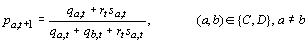[A.1]

Suppose, a was rewarded, i.e. sa,t ³ 0. Substitution of the response function r in [A.1] by rG with f = 0 yields, after some rearrangement, equation [A.2]: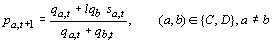.                                                    [A.2]

In equation [A.2], we substitute the terms corresponding to the right hand side of the probabilistic choice rule  by the choice probabilities in round t, pa,t  and pb,t = 1 - pa,t, respectively. Equation [A.2] then yields the new choice probability as a function of the old choice probability and the reinforcement. Equation [A.3] shows the rearrangement, where (a,b) Î {C,D} and a¹b: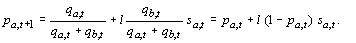[A.3]

The right hand side of [A.3] is equivalent to the BM updating of probabilities following “upward” reinforcement (or increase of propensity), defined in .

The BM equations for punishments of a are obtained in the same manner. Let sa,t  < 0. Then, substitution of the response function rG with f = 0 in equation [A.1] yields after some rearrangement the new probability for action a, given in [A.4]: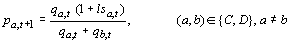[A.4]

Again, substitution of the terms for pa,t and pb,t = 1 - pa,t according to the probabilistic choice rule yields the new probability as the function of the preceding probability and the punishment that is specified by Equation [A.5].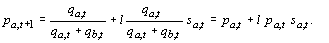[A.5]

The rightmost expression in equation [A.5] is identical with the BM stochastic learning rule for “downward” reinforcement (or reduction of propensity)  defined in .

We now show that the original Roth-Erev learning mechanism with response function rRE can also be obtained as a special case of a BM learning algorithm with a dynamic learning rate. Consider the case where action a was taken in t and rewarded (sa ³ 0). In the BM algorithm, the new probability pa,t+1 for action a is given by equation  above. To obtain the corresponding RE learning rule, we express the learning rate in the BM equation as a function of the present propensities and the most recent payoff. More precisely, the BM equation  expands to the RE equations  and  if the learning rate l is replaced as follows: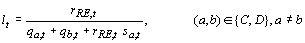.                                 [A.6]

Equation [A.6] shows that for rewards, the RE model is identical to the BM, with a learning rate that declines with the sum of the net payoffs for both actions in the past.

To obtain the BM equation for punishment, the corresponding learning rate function for punishment of action a is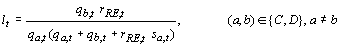[A.7]

Equation [A.7] modifies the learning rate as in [A.6], but it also ensures that the learning rate  is exponentially amplified as the propensity for the action taken approaches zero. This forces the behavior of the original RE model onto the BM equations.  Now the corresponding action propensity drops discontinuously down to its lower bound, a behavior that the BM model with a constant learning rate l avoids with the dampening term l sa pa., as pa moves towards zero. Conversely, [A.7] decreases the learning rate as the propensity for the alternative action b approaches zero. The latter effect of [A.7] obtains when the probability for action a is close to 1. In that case, the BM model with a constant learning rate l implies a rapid decline of the propensity of a after punishment, unlike the RE model. Equation [A.7] again forces RE behavior onto the BM equations, as the declining learning rate modifiesthe strong effect of punishment in this condition.

This paper is a modified version of an earlier publication: Flache, A, M.W. Macy (2002). The Power Law of Learning. Journal of Conflict Resolution 46(5):629-653. This research was made possible by a fellowship of the Royal Netherlands Academy of Arts and Sciences to the first author and the U.S. National Science Foundation (SES-0079381) to the second author. We also thank two anonymous reviewers, Yoshimichi Sato, Douglas Heckathorn, and other members of the Janus Workshop on Backward-Looking Rationality at Cornell University for their helpful suggestions. Please direct correspondence to the first author.

   Following Raub (1988, cf. Liebrand 1983), we use here a less restrictive criterion than Dawes (1980) originally proposed to define a social dilemma.

 In a Nash equilibrium, no one has an incentive to unilaterally change strategies, given the expected utility of the alternatives.

   The expected change in the probabilities of cooperation can also be zero when all outcomes are rewarding, but this equilibrium is not self-correcting. Rather, it is an unstable saddle point, from which any deviation will cause probabilities to move towards one of the self-reinforcing pure strategy equilibria of the game.

 The RE model also does not require that outcomes be normed to |s£ 1, but norming has no effect and simplifies comparison and integration of the two models.

 Habituation to repeated stimuli also affects the learning dynamics and can be modeled with a parameter that scales the degree to which aspiration levels float towards the mean of recent payoffs (Macy and Flache 2002; Erev et. al 1999). Macy and Flache (2002) explored the consequences of habituation using the Bush-Mosteller algorithm. In this paper, we focus on fixation and leave possible interactions with habituation for future research.

 Maximin is the largest possible payoff players can guarantee themselves in a social dilemma. This is P in PD and Stag Hunt and S in Chicken.

 Calibration of payoffs in decision tasks prior to a social dilemma game can be used to manipulate subjects’ aspiration levels. By setting R just above aspirations, we can test whether stochastic collusion obtains, as predicted by RE (due to the interaction with fixation) but not by Bush-Mosteller.